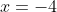## Example Questions

2 Next →

### Example Question #11 : Understanding Absolute Values

What is the sum of the two solutions to the equation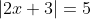?

-5

-3

-1

2

-3

Explanation:

To solve an equation with an absolute value, recognize that there are two things that would make an absolute value equal 5: the inside of the absolute value could equal 5, or it could equal -5. So your job is to solve for both possibilities:

Possibility 1: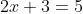You can then subtract 3 from both sides to get: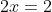And divide both sides by 2 to finish: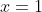Possibility 2: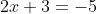Subtract 3 from both sides to get: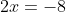Divide both sides by 2: